Most Affordable JEE | NEET | 8,9,10 Preparation by Kota's Top IITian Doctor Faculties

# Simple Harmonic Motion - JEE Advanced Previous Year Questions with Solutions`
JEE Advanced Previous Year Questions of Physics with Solutions are available at eSaral. Practicing JEE Advanced Previous Year Papers Questions of Physics will help the JEE aspirants in realizing the question pattern as well as help in analyzing weak & strong areas. Get detailed Class 11th & 12th Physics Notes to prepare for Boards as well as competitive exams like IIT JEE, NEET etc. eSaral helps the students in clearing and understanding each topic in a better way. eSaral is providing complete chapter-wise notes of Class 11th and 12th both for all subjects.       Download eSaral app  for free study material and video tutorials. Simulator Previous Years JEE Advanced Questions
Q. The x-t graph of a particle undergoing simple harmonic motion is shown below. The acceleration of the particle at t = 4/3 s is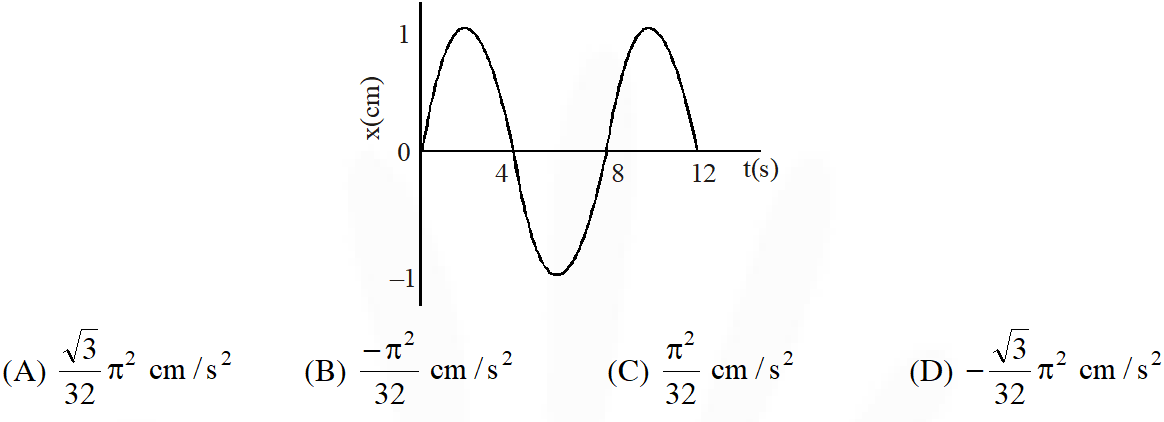[IIT JEE 2009]
Ans. (D) $\tau=8$ $\therefore \omega=\frac{\pi}{4}$ $\mathrm{x}=\sin \left(\frac{\pi}{4} \mathrm{t}\right)$ $\therefore a=-\frac{\pi^{2}}{16} \sin \left(\frac{\pi}{4} t\right)$ $-\frac{\pi^{2} \sqrt{3}}{32} \mathrm{cm} / \mathrm{s}^{2}$
Q. The mass M shown in the figure oscillates in simple harmonic motion with amplitude A. The amplitude of the point P is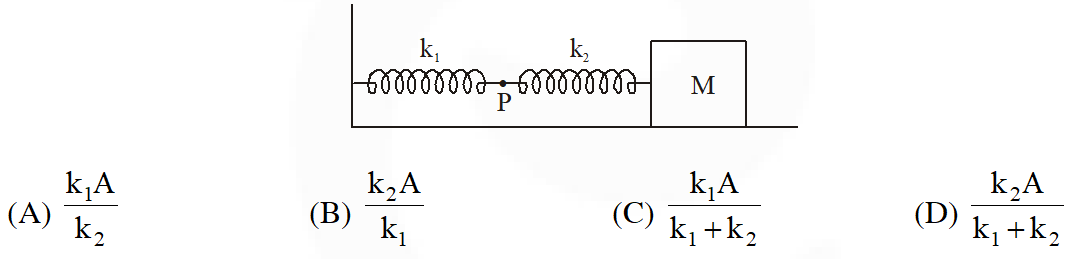[IIT JEE 2009]
Ans. (D) $\frac{\mathrm{k}_{1} \mathrm{k}_{2}}{\mathrm{k}_{1}+\mathrm{k}_{2}} \mathrm{A}=\mathrm{k}_{1} \mathrm{x}=\mathrm{F}$ $\therefore \mathrm{x}=\frac{\mathrm{k}_{2} \mathrm{A}}{\mathrm{k}_{1}+\mathrm{k}_{2}}$
Q. A uniform rod of length L and mass M is pivoted at the centre. Its two ends are attached to two springs of equal spring constants k. The springs are fixed to rigid supports as shown in the figure, and the rod is free to oscillate in the horizontal plane. The rod is gently pushed through a small angle $\theta$ in one direction and released. The frequency of oscillation is :-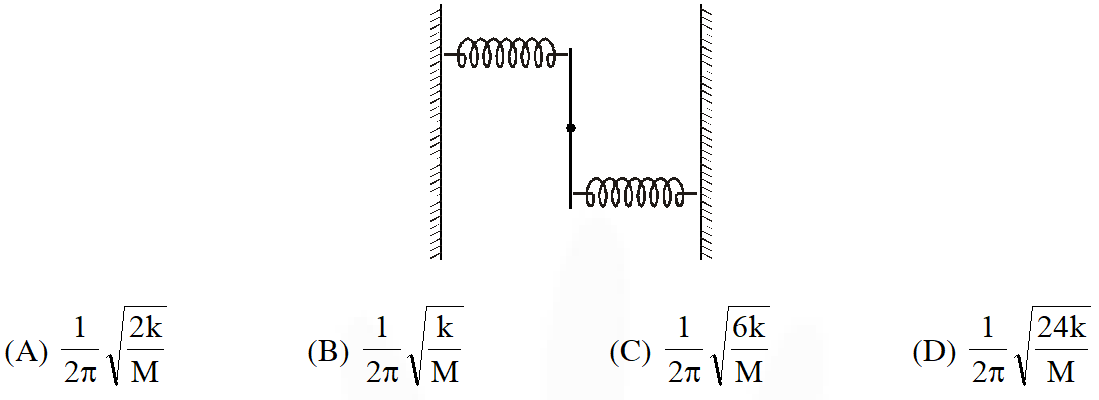[IIT JEE 2009]
Ans. (C) $\tau=2 \mathrm{k} \cdot \frac{\ell \theta}{2} \cdot \frac{\ell}{2}=\frac{\mathrm{m} \ell^{2}}{12} \cdot \omega^{2} \theta$ $\therefore \omega=\sqrt{\frac{6 \mathrm{k}}{\mathrm{m}}}$ $\therefore \mathrm{f}=\frac{1}{2 \pi} \sqrt{\frac{6 \mathrm{k}}{\mathrm{m}}}$
Paragraph for Question No. 4 to 6 When a particle of mass m moves on the x–axis in a potential of the form $\mathrm{V}(\mathrm{x})=\mathrm{k} \mathrm{x}^{2}$, it performs simple harmonic motion. The corresponding time period is proportional to $\sqrt{\frac{m}{k}}$, as can be seen easily using dimensional analysis. However, the motion of a particle can be periodic even when its potential energy increases on both sides of x = 0 in a way different from $\mathrm{kx}^{2}$ and its total energy is such that the particle does not escape to infinity. Consider a particle of mass m moving on the x–axis. Its potential energy is $\mathrm{V}(\mathrm{x})=\alpha \mathrm{x}^{4}(\alpha>0)$ for $|\mathrm{x}|$ near the origin and becomes a constant equal to $\mathrm{V}_{0}$ for $|\mathrm{x}| \geq \mathrm{X}_{0}$ (see figure)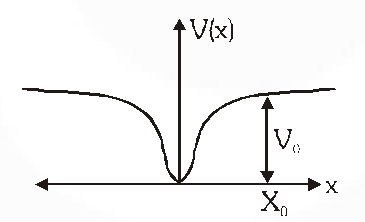Q. If the total energy of the particle is E, it will perform periodic motion only if :- (A) $\mathrm{E}<0$ (B) E > 0 (C) $\mathrm{V}_{0}>\mathrm{E}>0$ (D) $\mathrm{E}>\mathrm{V}_{0}$ [IIT-JEE 2010]
Ans. (C) Particle must be trapped. $\therefore \mathrm{V}_{0}>\mathrm{E}>0$
Q. For periodic motion of small amplitude A, the time period T of this particle is proportional to :- (A) $A \sqrt{\frac{m}{\alpha}}$ (B) $\frac{1}{A} \sqrt{\frac{m}{\alpha}}$ (C) $A \sqrt{\frac{\alpha}{m}}$ (D) $\frac{1}{A} \sqrt{\frac{\alpha}{m}}$ [IIT-JEE 2010]
Ans. (B) Dimentions are $\propto=\frac{\left[\mathrm{ML}^{2} \mathrm{T}^{-2}\right]}{\left[\mathrm{L}^{4}\right]}=\left[\mathrm{ML}^{-2} \mathrm{T}^{-2}\right]$ $\therefore \mathrm{T} \propto \frac{1}{\mathrm{A}} \sqrt{\frac{\mathrm{m}}{\alpha}}$
Q. The acceleration of this particle for $|x|>X_{0}$ is :- (A) proportional to $V_{0}$ (B) proportional to $\frac{V_{0}}{m X_{0}}$ (C) proportional to $\sqrt{\frac{V_{0}}{m X_{0}}}$ (D) Zero [IIT-JEE 2010]
Ans. (D) As $\mathrm{U}=\mathrm{V}_{0}=$ constant $\therefore \mathrm{a}=0$
Q. A point mass is subjected to two simultaneous sinusoidal displacements in x-direction, $x_{1}(t)=A \sin \omega t$ and $x_{2}(t)=A \sin \left(\omega t+\frac{2 \pi}{3}\right)$. Adding a third sinusoidal displacement $x_{3}(t)=B \sin (\omega t+\phi)$ brings the mass to a complete rest. The values of $\mathrm{B}$ and $\phi$ are :- [IIT-JEE 2011]
Ans. (B) $\mathrm{x}^{\prime}=\mathrm{A} \sin (\omega \mathrm{t})+\mathrm{A} \sin \left(\omega \mathrm{t}+\frac{2 \pi}{3}\right)$ $=2 \mathrm{A} \sin \left(\omega \mathrm{t}+\frac{\pi}{3}\right) \cos \left(\frac{\pi}{3}\right)$ $=\mathrm{A} \sin \left(\omega \mathrm{t}+\frac{\pi}{3}\right)$ $\therefore \mathrm{B}=\mathrm{A} \quad \phi=\frac{4 \pi}{3}$
Q. A metal rod of length ‘L’ and mass ‘m’ is pivoted at one end. A thin disk of mass ‘M’ and radius ‘R’ (< L) is attached at its center to the free end of the rod. Consider two ways the disc is attached: (case A). The disc is not free to rotate about its center and (case B) the disc is free to rotated about its center. The rod-disc system performs SHM in vertical plane after being released from the same displaced position. Which of the following statement(s) is(are) true?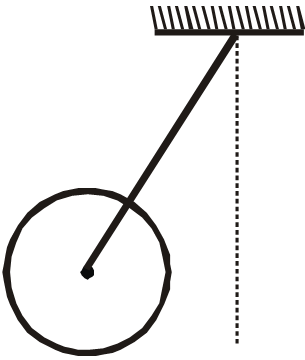(A) Restoring torque in case A = Restoring torque in case B (B) Restoring torque in case A < Restoring torque in case B (C) Angular frequency for case A> Angular frequency for case B (D) Angular frequency for case A< Angular frequency for case B [IIT-JEE 2011]
Ans. (A,D) $\tau=\frac{\mathrm{mL}}{2} \mathrm{g} \cos \theta+\mathrm{mL} \mathrm{g} \cos \theta \mathrm{same}$ $\frac{\omega}{2 \pi}=\mathrm{f}$ for $\mathrm{A} \mathrm{mg} \mathrm{H}=\frac{1}{2} \mathrm{mv}^{2} \&$ for $\mathrm{B}=\operatorname{mg} \mathrm{x}=\frac{1}{2} \mathrm{mv}^{2}+\frac{1}{2} \mathrm{I} \omega^{2}$ $\therefore \omega$
Q. A small block is connected to one end of a massless spring of un-stretched length 4.9 m. The other end of the spring (see the figure) is fixed. They system lies on a horizontal frictionless surface. The block is stretched by 0.2 m and released from rest at t = 0. It then executes simple harmonic motion with angular frequency $\omega=\frac{\pi}{3}$ rad/s. Simultaneously at t = 0, a small pebble is projected with speed v from point P at an angle of 45° as shown in the figure. Point P is at a horizontal distance of 10m from O. If the pebble hits the block at t = 1s, the value of v is:- (take $\left.\mathrm{g}=10 \mathrm{m} / \mathrm{s}^{2}\right)$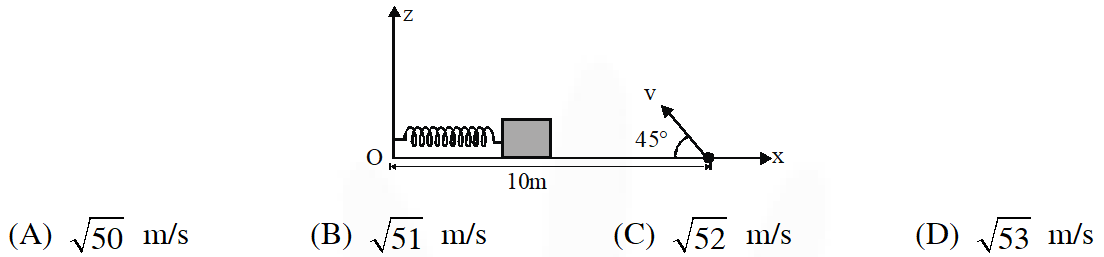[IIT-JEE 2012]
Ans. (A) Time of $\therefore \mathrm{v}=\frac{\mathrm{g}}{\sqrt{2}}=\sqrt{50} \mathrm{m} / \mathrm{s}$
Q. A particle of mass m is attached to one end of a mass-less spring of force constant k, lying on a frictionless horizontal plane. The other end of the spring is fixed. The particle starts moving horizontally from its equilibrium position at time t = 0 with an initial velocity $\mathbf{u}_{0}$. When the speed of the particle is 0.5 $\mathbf{u}_{0}$, it collides elastically with a rigid wall. After this collision :- (A) the speed of the particle when it returns to its equilibrium position is $\mathrm{u}_{0}$ (B) the time at which the particle passes through the equilibrium position for the first time is $t=\pi \sqrt{\frac{m}{k}}$ (C) the time at which the maximum compression of the spring occurs is $\mathrm{t}=\frac{4 \pi}{3} \sqrt{\frac{\mathrm{m}}{\mathrm{k}}}$ (D) the time at which the particle passes through the equilibrium position for the second time is $t=\frac{5 \pi}{3} \sqrt{\frac{m}{k}}$ [JEE-Advanced-2013]
Ans. (A,D) Collision is elastic so energy is conserve in given question so when it return to equilibrium, its speed is $\mathrm{u}_{0}$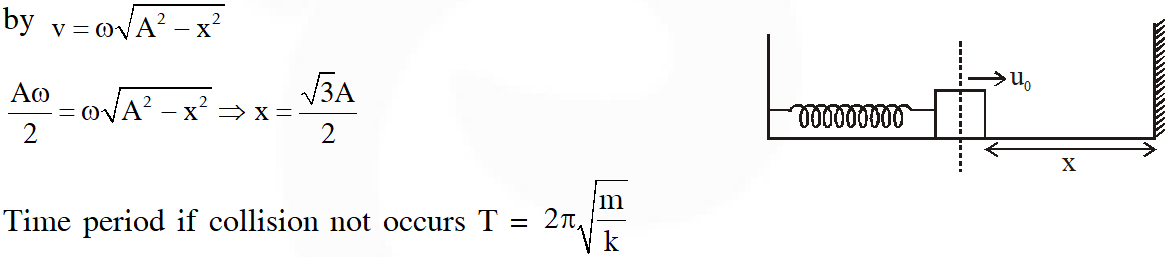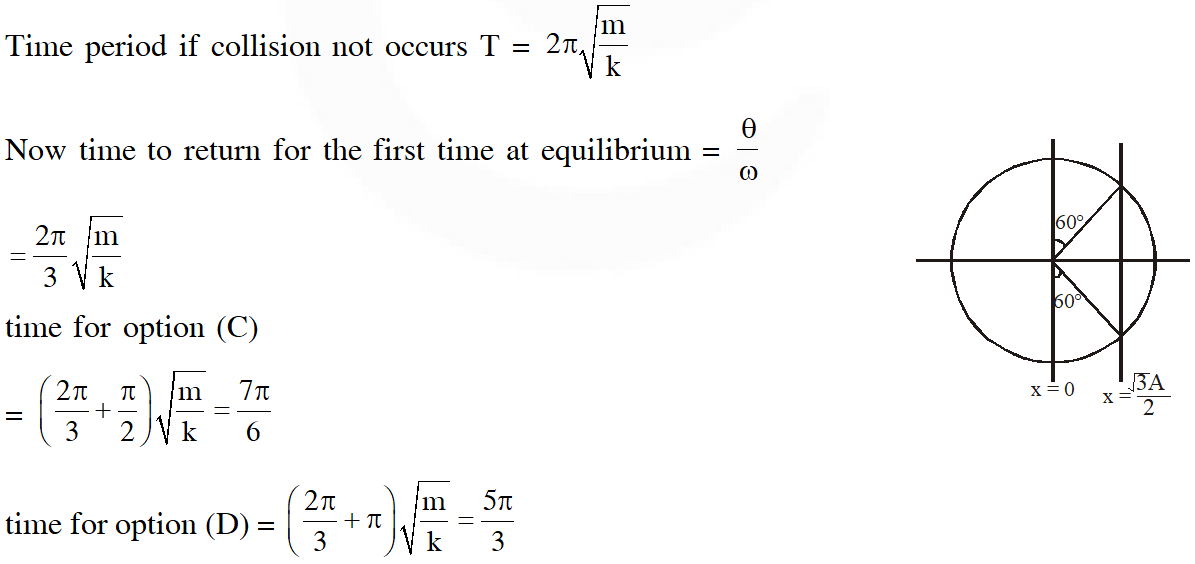Q. Two independent harmonic oscillators of equal mass are oscillating about the origin with angular frequencies  and have total energies $\mathrm{E}_{1}$ and $\mathrm{E}_{2}$, respectively. The variations of their momenta p with positions x are shown in the figures. If $\frac{\mathrm{a}}{\mathrm{b}}=\mathrm{n}^{2}$ and $\frac{\mathrm{a}}{\mathrm{R}}=\mathrm{n}$, then the correct equation (s) is (are)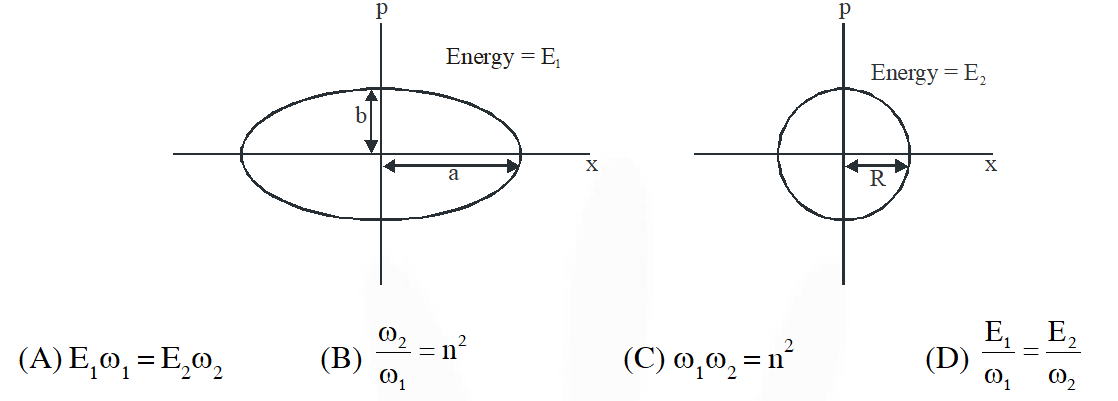[JEE-Advanced-2015]
Ans. (B,D) $\mathrm{P}_{1 \max }=\operatorname{mac}_{1}=\mathrm{b}$ $\mathrm{P}_{2 \max }=\mathrm{mR} \omega_{2}=\mathrm{R}$ $\frac{\omega_{1}}{\omega_{2}}=\frac{1}{n^{2}}$ $\frac{\omega_{2}}{\omega_{1}}=\mathrm{n}^{2}$ $\mathrm{E}_{1}=\frac{1}{2} \mathrm{m} \omega_{1}^{2} \mathrm{a}^{2}$
Q. A particle of unit mass is moving along the x-axis under the influence of a force and its total enegy is conserved. Four possible forms of the potential energy of the particle are given in column I (a and $\mathrm{U}_{0}$ are constants). Match the potential energies in column I to the corresponding statement(s) in column-II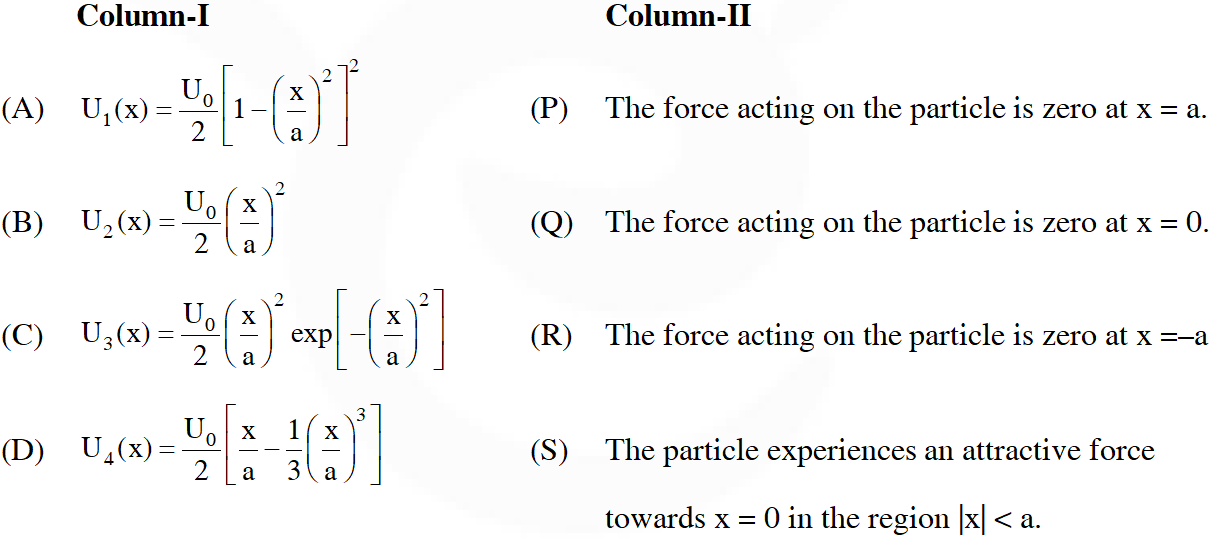[JEE-Advanced-2015]
Ans. ($(\mathrm{A})-\mathrm{P}, \mathrm{Q}, \mathrm{R}, \mathrm{T} ;(\mathrm{B})-\mathrm{Q}, \mathrm{S} ;(\mathrm{C})-\mathrm{P}, \mathrm{Q}, \mathrm{R}, \mathrm{S} ;(\mathrm{D})-\mathrm{P}, \mathrm{R}, \mathrm{T}$)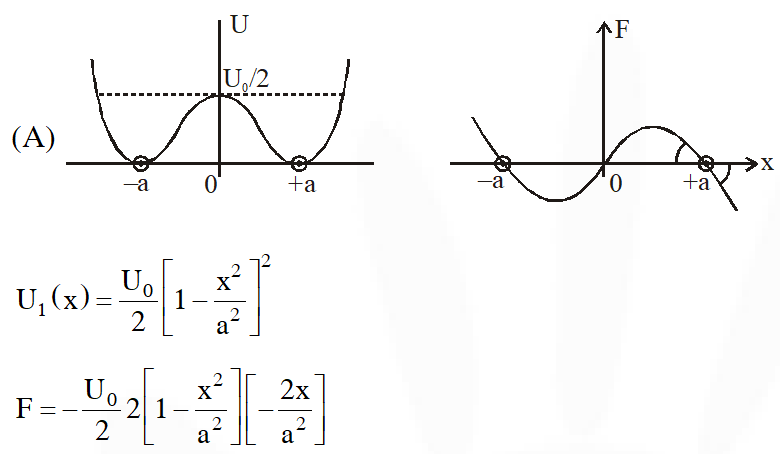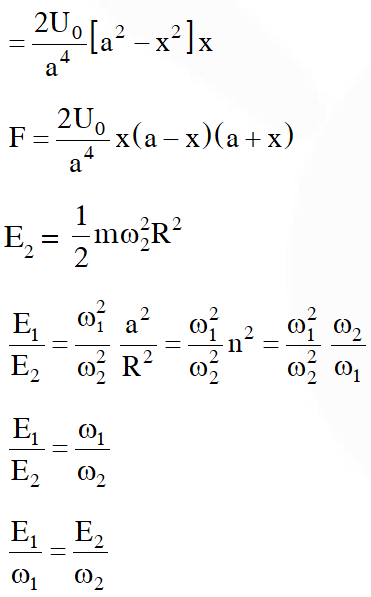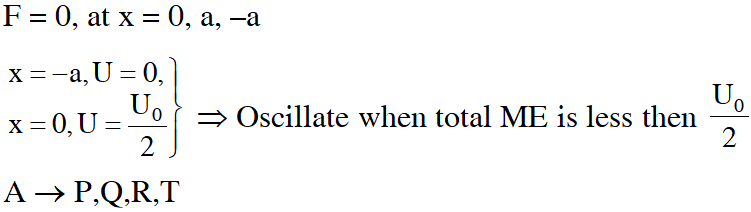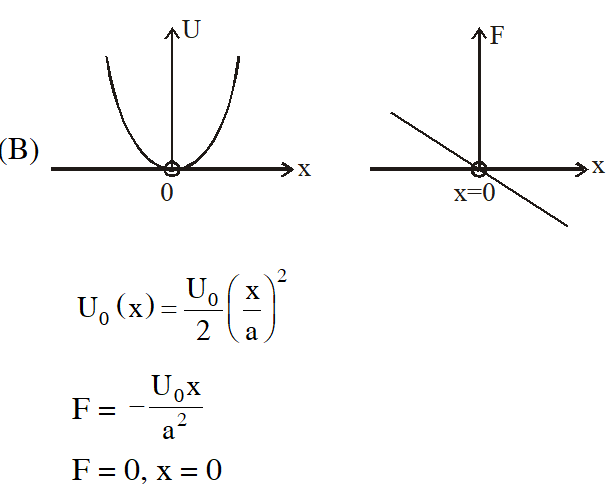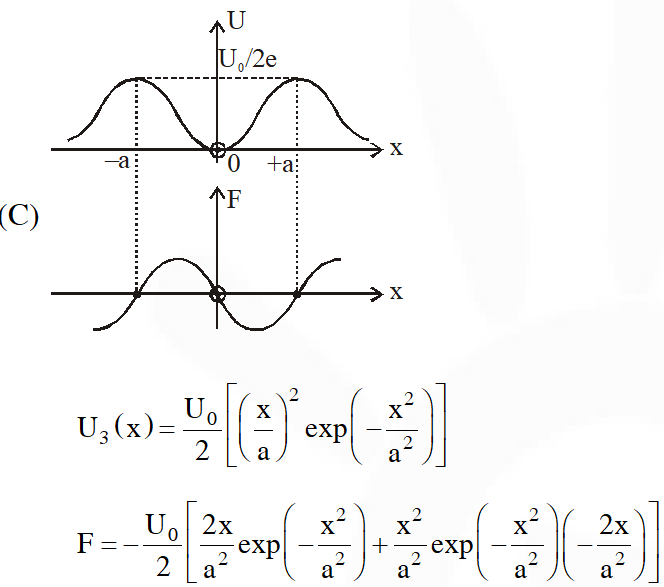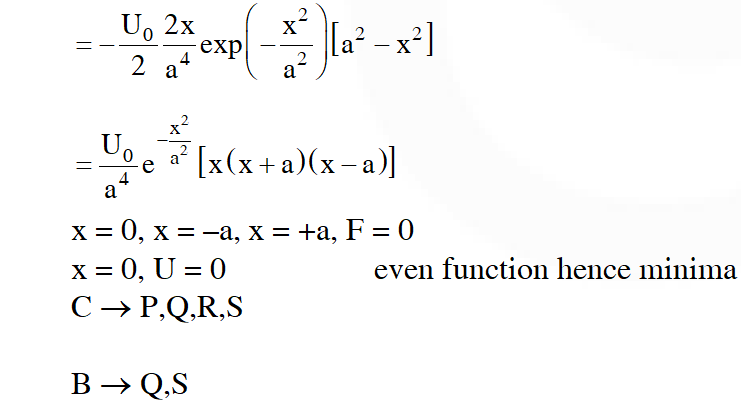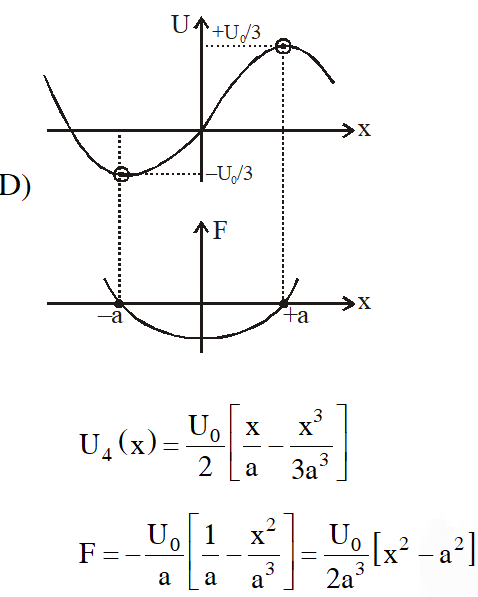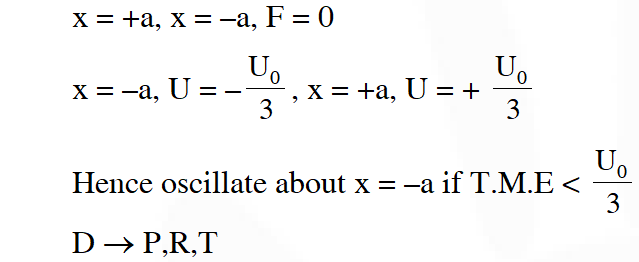Q. A block with mass M is connected by a massless spring with stiffness constant k to a rigid wall and moves without friction on a horizontal surface. The block oscillates with small amplitude A about an equilibrium position x0. Consider two cases : (i) when the block is at x0; and (ii) when the block is at x = x0 + A. In both the cases, a particle with mass m ( (A) The amplitude of oscillation in the first case changes by a factor of , whereas $\sqrt{\frac{\mathrm{M}}{\mathrm{m}+\mathrm{M}}}$ in the second case it remains unchanged (B) The final time period of oscillation in both the cases is same (C) The total energy decreases in both the cases (D) The instantaneous speed at x0 of the combined masses decreases in both the cases. [JEE-Advanced-2016]
Ans. (A,B,D) $\mathrm{T}_{\mathrm{i}}=2 \pi \sqrt{\frac{\mathrm{M}}{\mathrm{K}}}, \mathrm{T}_{\mathrm{f}}=2 \pi \sqrt{\frac{\mathrm{M}+\mathrm{m}}{\mathrm{K}}}$ case (i) : M $(\mathrm{A} \omega)=(\mathrm{M}+\mathrm{m}) \mathrm{V}$ $\therefore$ Velocity decreases at equilibrium position. By energy conservation $\mathrm{A}_{\mathrm{f}}=\mathrm{A}_{\mathrm{i}} \sqrt{\frac{\mathrm{M}}{\mathrm{M}+\mathrm{m}}}$ case ( ii ) : No energy loss, amplitude remains same At equilibrium $\left(\mathrm{x}_{0}\right)$ velocity $=\mathrm{A} \omega .$ In both cases $\omega$ decreases so velocity decreases in both cases
Q. Two vectors $\overrightarrow{\mathrm{A}}$ and $\overrightarrow{\mathrm{B}}$ are defined as $\overrightarrow{\mathrm{A}}=\overrightarrow{\mathrm{A}}=$ ai and $\overrightarrow{\mathrm{B}}=\mathrm{a}(\cos \omega \mathrm{ti}+\sin \omega \mathrm{t} \hat{\mathrm{j}}),$ whre a is a constant and $\omega=\pi / 6 \mathrm{rad} \mathrm{s}^{-1} .$ If $|\overrightarrow{\mathrm{A}}+\overrightarrow{\mathrm{B}}|=\sqrt{3}|\overrightarrow{\mathrm{A}}-\overrightarrow{\mathrm{B}}|$ at time $\mathrm{t}=\tau$ for the first time, the value of $\tau,$ in seconds, is [JEE-Advanced-2018]
Ans. 2.00 Sec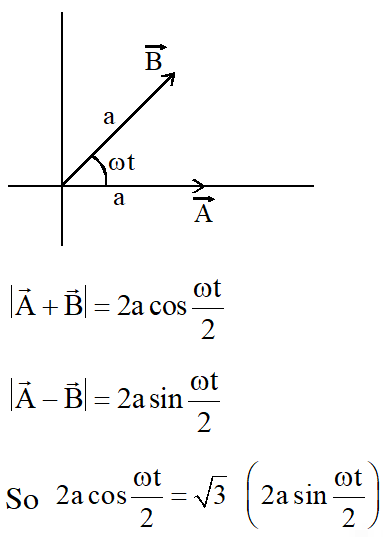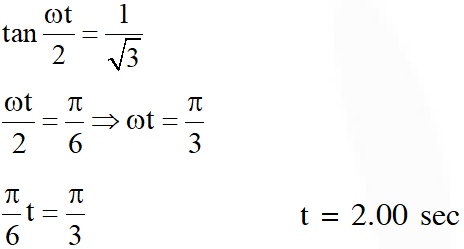Sonu
May 6, 2023, 6:35 a.m.
Thnx
Sonu
May 6, 2023, 6:35 a.m.
Thnx
Lame
March 30, 2022, 5:01 p.m.
Lame
Chirag falor
Oct. 1, 2021, 6:25 p.m.
simple hamronic mothion
hhghfy
April 5, 2021, 6:48 p.m.
hi
Superstar rajinikanth
Dec. 11, 2020, 6:25 a.m.
Dengey
x
Sept. 18, 2020, 7:10 p.m.